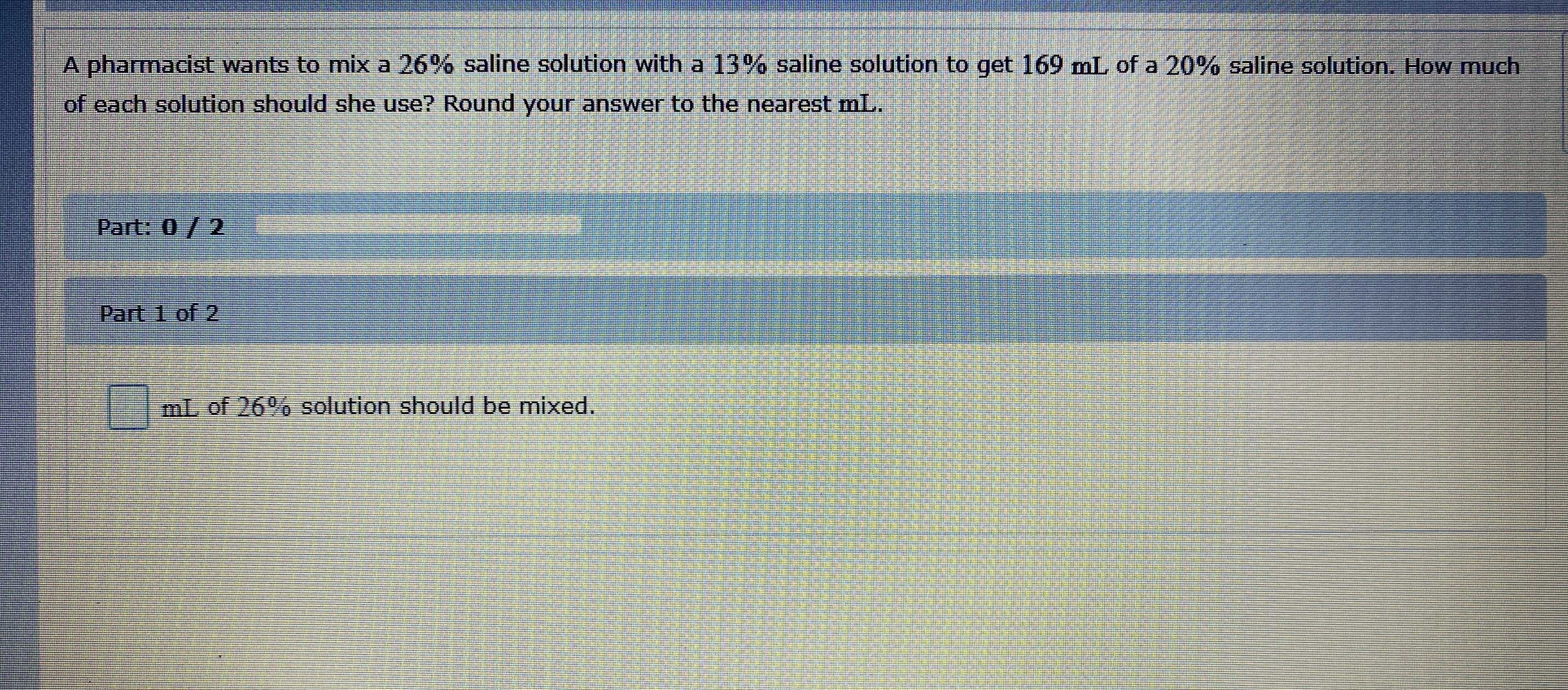### ¿Todavía tienes preguntas de matemáticas?

Pregunte a nuestros tutores expertos
Algebra
PreguntaA pharmacist wants to mix a $$26 \%$$ saline solution with a $$13 \%$$ saline solution to get $$169 mL$$ of a $$20 \%$$ saline solution. How much of each solution should she use? Round your answer to the nearest mL.

Part $$1$$ of $$2$$

$$\square$$mL of $$26 \%$$ solution should be mixed.

$$91$$